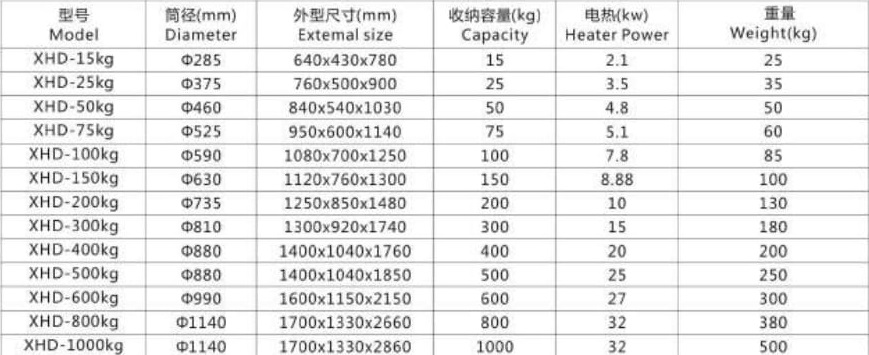Phone Number

# XHD Standard Hopper dryer

## XHD Standard Hopper dryer

Save time

Save manpower

Air filter

Magnet base

Model:

XHD-15KG (W=25KG)

XHD-25KG (W=35KG)

XHD-50KG (W=50KG)

XHD-75KG (W=60KG)

XHD-100KG (W=85KG)

XHD-150KG (W=100KG)

XHD-200KG (W=130KG)

XHD-300KG (W=180KG)

XHD-400KG (W=200KG)

XHD-500KG (W=250KG)

XHD-600KG (W=300KG)

XHD-800KG (W=380KG)

XHD-1000KG (W=500KG)